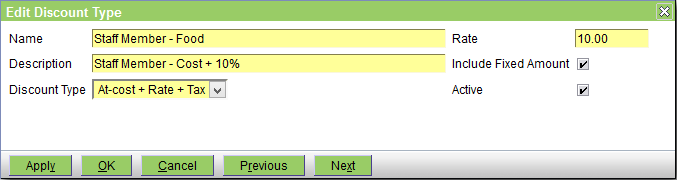# Discount

This screen allows you to create/view/edit the Discount Types. See also Concepts|Discounts.The fields are as follows:
Name - the name of the discount
Description - a description that serves to clarify the name
Discount Type - either Percentage (ie a percentage of the amount), Fixed (ie a fixed amount), or At-cost + Rate + Tax
Rate - either the fixed amount (for the Fixed type), or the percentage amount (for the Percentage and At-cost types)
Include Fixed Amount - check this box if the discount is to apply to the fixed component of the product price as well as its unit component
Active - uncheck this box to deactivate the discount

Note that the 'At-cost + Rate + Tax' type is so-named to reflect how the discount amount is calculated. Whereas with a Percentage discount of 10%, the sale price is reduced by 10% and thus the discount amount is 10% of the original sale price.

With an At-cost discount of 10%, the item is sold for (cost+10%)+tax, and thus the discount amount will depend on the markup.